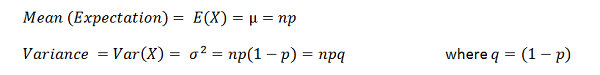# How to Calculate Binomial Probability

Binomial distribution is one of the elementary probability distributions for discrete random variables used in probability theory and statistics. It is given the name because it has the binomial coefficient that is involved in every probability calculation. It weighs in the number of possible combinations for each configuration.

Consider a statistical experiment with each event having two possibilities (success or failure) and p probability of success. Also, each event is independent from one another. A single event of such nature is known as a Bernoulli trial. Binomial distributions are applied to successive sequence of Bernoulli trials. Now, let us take a look at the method to find binomial probability.

## How to Find Binomial Probability

If X is the number of successes from n (finite amount) independent Bernoulli trials, with the probability of success p, then the probability of X successes in the experiment is given by,

nCx is called the binomial coefficient.

X is said to be binomially distributed with parameters p and n, often denoted by the notation Bin(n,p).

The Mean and the variance of the Binomial distribution are given in terms of the parameters n and pThe shape of the Binomial distribution curve also depends on the parameters n and p. When n is small, the distribution is roughly symmetric for values p≈.5 range and highly skewed when p is in 0 or 1 range. When n is large, the distribution becomes more smoothed and symmetric with noticeable skew when p is in the extreme 0 or 1 range. In the following diagram, x-axis represents the number of trials and y axis gives the probability.## How to Calculate Binomial Probability – Examples

1. If a biased coin is tossed 5 times successively and the chance of success is 0.3, find the probabilities in following instances.

a) P(X=5)                     b) P(X) ≤ 4                         c) P(X) < 4

d) Mean of the distribution

e) Variance of the distribution

From the details of the experiment we can deduce that the distributions of probabilities are binomial in nature with 5 successive and independent trials with success probability 0.3.Therefore n=5 and p=0.3.

a) P(X=5) = probability of getting successes (heads)for all five trials

P(X=5) = 5C5 (0.3)5 (1 – 0.3)5 – 5 = 1 × (0.3)5 × (1) = 0.00243

b) P(X) ≤ 4 = probability of getting four or less number of successes during the experiment

P(X) ≤ 4 = 1-P(X=5) = 1-0.00243 = 0.99757

c)  P(X) < 4 = probability of getting less than four successes

P(X) < 4 = [P(X=1) + P(X=2) + P(X=3)] = 1- [P(X=4) + P(X=5)]

To calculate binomial probability of getting only four success (P(X)=4) we have,

P(X=4) = 5C4 (0.3)4 (1 – 0.3)5-4 = 5×0.0081×(0.7) = 0.00563

P(X) < 4 = 1 – 0.00563 – 0.00243 = 0.99194

d)  Mean = np = 5(0.3) = 1.5

e) Variance = np(1 – p) = 5(0.3)(1-0.3) = 1.05